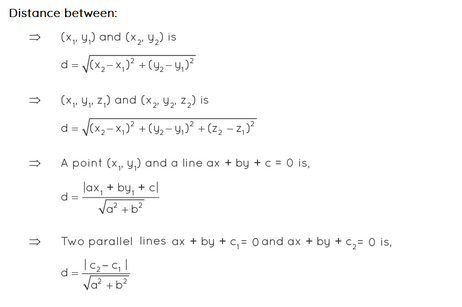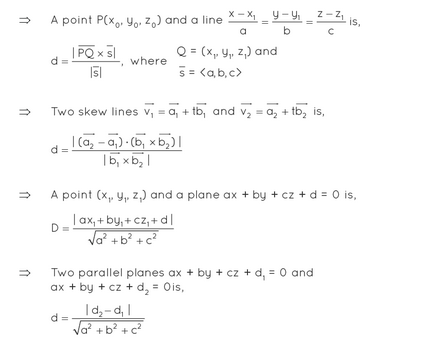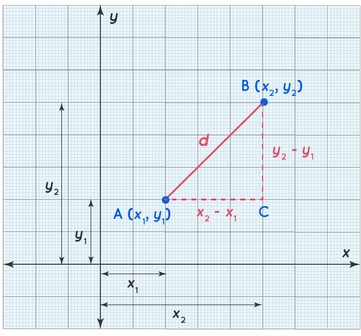Distance Formula homework Help at TutorEye

# Best Homework Help For Distance Formula

## Top Questions

E.g. 5% charge on every 10% breach of the total breach of 102%. Any short summed-up formula?
View More

How far did he run on Tuesday? Enter your answer in the box as a fraction in simplest form.
View More

ou’ll be using the same 4 numbers written in the same order. The only thing that will change in each expression will be the tools you’ll use from your toolbox. The tools that you’ve learned so far for order of operations are parentheses, exponents, multiplying, dividing, adding, and subtracting. Here are the four numbers. Keep them in this order: 18 2 4 3 Here are the tools in your toolbox: The 2 and 3 represent exponents, so you are allowed to square or cube a number. Listed below are the values you need to create. 21 = 67 = -6 = 103 =
View More

profit of 20%. If his gain on the sale of one electronic item is rupees 4500 find the Marked price of the electronic item.
View More

## Distance Formula:

The length of the line connecting two places is the distance between them.And the formula which is used to derive its length is called distance formula.

If the two locations are on the same horizontal or vertical line, the distance between them can be calculated by subtracting the non-overlapping coordinates.

## Distance Formula Frequently Asked Questions:

Question 1: What is the distance formula?

The length of the line segment connecting two points is equal to their distance.The Pythagoras theorem is used to obtain the formula for the distance between two points in a two-dimensional plane, which may also be used to estimate the distance between two points in a three-dimensional plane.

Question 2: What is the distance formula?

In coordinate geometry, there are several different forms of distance formulas which are listed below:Question 3: What is the distance formula?

Let us assume the points A(x1, y1) and B(x2, y2)

Let ‘d’ be the distance between A and B.

By using pythagoras theorem

AB2 = AC2 + BC2

d2 = (x- x1) + (y-  y1)

d = √(x- x1) + (y-  y1)Question 4: What is the distance formula?

A(5,2) and B(8,11)

AB2 = (8-5)2 + (11-2)2

AB = √(3)2 + (9)2 = √9 + 81 = 3√10